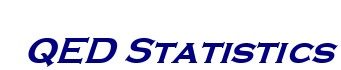Regression: mapping the correlation between samples

The Regression menu gives 5 powerful and customisable regression analyses:

• Pearson Correlation - the most widely used measure of correlation or association between two variables.
• Kendall Correlation - non-parametric correlation measure between two variables.
• Spearman Rank Correlation - measures the direction and strength of the relationship between two variables.
• Linear Regression - to fit a linear equation to two variables.
• Multiple Linear Regression - to fit a linear equation with two or more independent variables.You can set up a regression analysis with just 3 mouse-clicks.